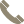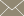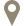Home » Faculty Profiles » Faculty

### Lin, Runchang

Professor

#### CONTACT INFO(956) 326-2569rlin@tamiu.eduLBV-317

#### COURSES TAUGHT

• Advanced Topics in Mathematics (MATH 5355)
• Combinatorics (MATH 5315)
• Complex Variables (MATH 4345)
• Complex Variables I (MATH 5320)
• Functional Analysis I (MATH 5312)
• Linear Algebra (MATH 5306)
• Mathematical Modeling (MATH 5370)
• Number Theory (MATH 4305)
• Number Theory I (MATH 5303)
• Number Theory II (MATH 5304)
• Numerical Analysis I (MATH 4340)
• Ordinary Diff Equations (MATH 3330)
• Ordinary Diff Equations I (MATH 5350)
• Ordinary Differential Equation (MATH 3330)
• Partial Differential Equations (MATH 5360)
• Real Analysis I (MATH 5305)
• Selected Topics in Math (MATH 4355)
• Senior Mathematics Project (MATH 4395)
• Thesis I (MATH 5398)
• Thesis II (MATH 5399)